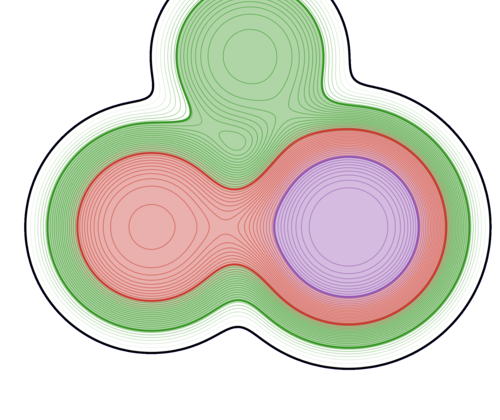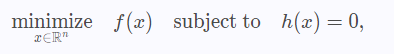## Julia Community 🟣# New JSO package: DCISolver.jl

We are very happy to announce the publication in the Journal of Open Source Software of the paper DCISolver.jl: A Julia Solver for Nonlinear Optimization using Dynamic Control of Infeasibility. It is accessible in open access here.

`DCISolver.jl` is a new pure Julia implementation of the Dynamic Control of Infeasibility method (DCI), introduced by Bielschowsky and Gomes in 2008, for solving the equality-constrained nonlinear optimization problemwhere \$f\$ and \$h\$ are twice continuously differentiable.
DCI is an iterative method that aims to compute a local minimum using first and second-order derivatives.
Our initial motivation for developing `DCISolver.jl` is to solve PDE-constrained optimization problems, many of which have equality constraints only.

`DCISolver.jl` is built upon the JuliaSmoothOptimizers (JSO) tools, and takes as input an `AbstractNLPModel`, JSO's general model API defined in `NLPModels.jl`, a flexible data type to evaluate objective and constraints, their derivatives, and to provide any information that a solver might request from a model. The user can hand-code derivatives, use automatic differentiation, or use JSO-interfaces to classical mathematical optimization modeling languages such as AMPL, CUTEst, or JuMP.

We refer to the documentation and tutorials for examples and benchmarks.

## Example

In the following example, we use `DCISolver.jl` on two optimization problems modeled with `ADNLPModels.jl` using automatic differentiation to compute the derivatives.

``````using DCISolver, ADNLPModels
# Unconstrained Rosenbrock optimization
nlp = ADNLPModel(x -> 100 * (x - x^2)^2 + (x - 1)^2, [-1.2; 1.0])
stats = dci(nlp)
# Constrained optimization
nlp = ADNLPModel(x -> 100 * (x - x^2)^2 + (x - 1)^2, [-1.2; 1.0],
x->[x * x - 1], [0.0], [0.0])
stats = dci(nlp)
``````

## References

Migot, T., Orban, D., & Siqueira, A. S.
DCISolver. jl: A Julia Solver for Nonlinear Optimization using Dynamic Control of Infeasibility.
Journal of Open Source Software, 7(70), 3991 (2022).
10.21105/joss.03991

Bielschowsky, R. H., & Gomes, F. A.
Dynamic control of infeasibility in equality constrained optimization.
SIAM Journal on Optimization, 19(3), 1299-1325 (2008).
10.1007/s10589-020-00201-2